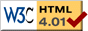# What Is A Differential Equation?

previous next

A differential equation can look pretty intimidating, with lots of fancy math symbols. But the idea behind it is actually fairly simple:

A differential equation states how a rate of change (a "differential") in one variable is related to other variables.

For example, the Single Spring simulation has two variables: the position of the block, x , and its velocity, v . Each of those variables has a differential equation saying how that variable evolves over time. The differential equation for position x is

x' = v

where x' indicates the derivative of x with respect to time (the rate of change of x ). This equation says

the rate of change of position is equal to the velocity

That's sort of an obvious statement. Hang on, the next equation is more interesting:

The amount of stretch in the spring is directly related to the position of the block, x . You can see the details on the Single Spring simulation page, but using Newton's Law F = m a we are able to write the differential equation for the velocity v as

v' = −k x

where k is the spring constant (how stiff the spring is). This equation says

the rate of change of velocity is inversely proportional to the position

For instance, when the position is zero (ie. the spring is neither stretched nor compressed) then the velocity is not changing. This makes sense, because the spring is not exerting a force at that moment.

On the other hand, when the position is large (ie. the string is very much stretched or compressed) then the rate of change of the velocity is large, because the spring is exerting a lot of force.

## What is a Solution to a Differential Equation?

When you begin learning mathematics, you work on getting solutions to equations like

x2 + 2 x + 1 = 0

which has a solution x = −1 For a differential equation, the solution is not a single value, but a function. The task is to find a function whose various derivatives fit the differential equation over a long span of time. For example,

 x'' + 2 x' + x = 0 (1)

is a differential equation where the goal is to find a function x(t) which, when you plug the function and its derivatives into the differential equation, the equation holds for any time t .

The general solution for the previous equation happens to be

 x(t) = a e−t + b t e−t (2)

where e=2.71828... and a, b are undetermined constants. It is easy to confirm that you have a solution: just plug the solution in to the differential equation! For our example, we find the first and second derivatives (see the math refresher for how to find these derivatives... it's pretty easy!):

 x'(t) = (b − a)e−t − b t e−t (3)
 x''(t) = (a − 2 b)e−t + b t e−t (4)

Now plug these equations (2), (3), and (4) into the left side of the differential equation (1), and do the algebra:

x'' + 2 x' + x =

= ((a − 2 b)et + b t et) + 2((ba)etb t et) + (a et + b t et)

= (a − 2 b + 2 b − 2 a + a)et + (b − 2 b +b) t et

= 0

Therefore the solution (2) satisfies the differential equation (1) for any values of a, b .

The solution is called the general solution because we have not yet applied a particular set of initial conditions.

## Initial Conditions

In the above example we are left with undetermined constants a, b . How do we find out what they are? They are set according to the initial conditions which are the particular starting values of the variables. For example, with the Single Spring simulation the initial conditions are the starting position and velocity of the block at time t = 0 .

For the example problem above, we might have initial conditions specifying position x and velocity x' at time t = 0 as follows

x(0) = 1
x'(0) = 0

Then we can plug t = 0 into equations (2) and (3) above to find the values of the constants a, b

x(0) = a + 0 = 1

x'(0) = (ba) − 0 = 0

and therefore we find that a = b = 1 and the particular solution is

x(t) = et + t et

This solution is called the particular solution because it applies only to the particular initial conditions that we have chosen.

previous next